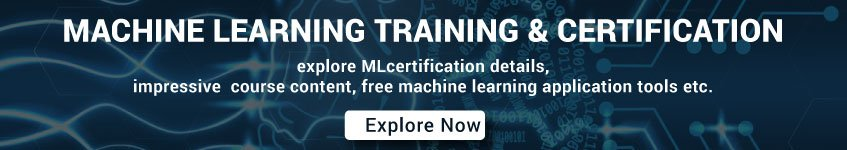# Machine Learning Interview Questions and Answers Set 8

71. What are the three stages to build the hypotheses or model in machine learning?

1. Model building
2. Model testing
3. Applying the model

72. What is the standard approach to supervised learning?

The standard approach to supervised learning is to split the set of example into the training set and the test.

73. What is ‘Training set’ and ‘Test set’?

In various areas of information science like machine learning, a set of data is used to discover the potentially predictive relationship known as ‘Training Set’. The training set is an example given to the learner, while Test set is used to test the accuracy of the hypotheses generated by the learner, and it is the set of example held back from the learner. The training set is distinct from the Test set.

74. List down various approaches to machine learning?

The different approaches in Machine Learning are

i) Concept Vs Classification Learning

ii) Symbolic Vs Statistical Learning

iii) Inductive Vs Analytical Learning

75. What is not Machine Learning?

i) Artificial Intelligence

ii) Rule-based inference

76. Explain what is the function of ‘Unsupervised Learning’?

1. Find clusters of the data
2. Find low-dimensional representations of the data
3. Find interesting directions in data
4. Interesting coordinates and correlations
5. Find novel observations/ database cleaning

77. Explain what is the function of ‘Supervised Learning’?

1. Classifications
2. Speech recognition
3. Regression
4. Predict time series
5. Annotate strings

78. What is algorithm independent machine learning?

Machine learning in where mathematical foundations are independent of any particular classifier or learning algorithm is referred to as algorithm independent machine learning?

79. What is the difference between artificial learning and machine learning?

Designing and developing algorithms according to the behaviors based on empirical data are known as Machine Learning.  While artificial intelligence in addition to machine learning, it also covers other aspects like knowledge representation, natural language processing, planning, robotics etc.

80. What is the classifier in machine learning?

A classifier in a Machine Learning is a system that inputs a vector of discrete or continuous feature values and outputs a single discrete value, the class.